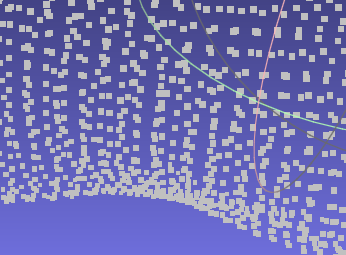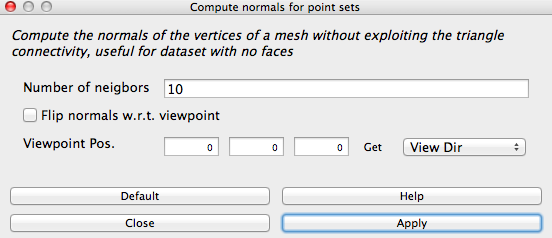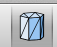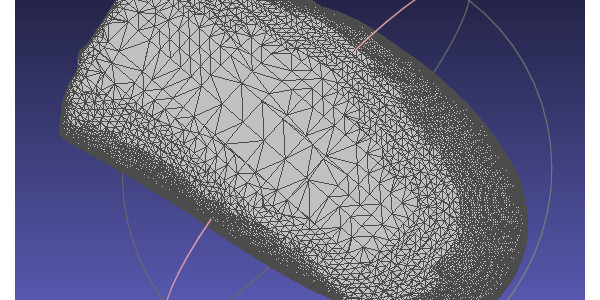# How to Create an STL file from a XYZ file in MeshLab

"MeshLab is an opensource, portable, and extensible system for the processing and editing of unconstructed 3D triangular meshes." This tutorial shows the workflow from how to import an XYZ file and export an STL file. The input could be from a laser scanner, and the output is meant for a 3D printer.

• Step 1: File Import XYZ
• Step 2: Create Normals and Mesh
• Step 3: Export to STL
• Resources

### Step 1: File Import Mesh

1. Start MeshLab.
2. File -> Import Mesh.
3. Select the *.XYZ file.

Now you should see the point cloud similar to the image below.If you zoom up, you will see it consists of a lot of points like the image below.### Step 2: Create Normals and Mesh

1. Click on Filters -> Normals, Curvatures and Orientation -> Compute Normals for Point Sets.
2. The window below will appear. As stated, this function is good for meshing without exploiting the triangle connectivity and is useful for datasets without faces. The 10 represents the number of points used to estimate the tangent plane at each point set. From this, normals are calculated for the mesh. In my lab experience, 10 neighbors is a good number.
3.4. Click Apply to begin computing. This may take a little time.
5. Click Filters -> Remeshing, Simplification and Reconstruction -> Surface Reconstruction: Poisson. Poisson is a common surface reconstruction algorithm. It takes the normals from each point to calculate the surface. The parameters I used in this example are below. Play around with the numbers. NOTE: If the Octree Depth is large, it may crash your computer.
6.7. Click Apply, again the computer is working overtime and calculating many equations so it may take some time.
8. Show flat lines by pressing the icon on the toolbar.NOTE: Notice the holes are gone and the surface is filled with triangles.### Step 3: Export to STL

1. File -> Export Mesh as STL File